Volume 4, Issue 5-1, October 2015, Pages: 15-29

Variability in Mechanical Properties of Agave Americana L. Fiber "Intra-Plant Study"

Asma El Oudiani1, Slah Msahli1, Faouzi Sakli1, Jean Yves Drean2

1Laboratory of Textile Engineering, University of Monastir, ISET Ksar Hellal, B.P 68 Ksar Hellal, Tunisia

2Laboratory of Textile Physics and Mechanics, ENSITM, 11 rue Alfred Werner, Mulhouse Cedex, France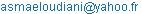(A. E. Oudiani)

Asma El Oudiani, Slah Msahli, Faouzi Sakli, Jean Yves Drean. Variability in Mechanical Properties of Agave Americana L. Fiber "Intra-Plant Study". Advances in Materials. Special Issue: New Methods of Extraction and Characterization of Plant Fibers. Vol. 4, No. 5-1, 2015, pp. 15-29. doi: 10.11648/j.am.s.2015040501.13

Abstract: In this work, a study of intra plant variability in agave fiber mechanical properties was conducted. It is based on the Design of experiments techniques and statistical analyses. By taking into account the variation of modalities related to the fiber collecting mode, some parameters were defined and considered as controlling factors, namely the position of the fiber in the leaf (fiber maturity) and the level of the leaf in the plant (leaf age). The main results show that fibers taken from different leaves at the same level of a plant have approximately much close properties. However, fibers located at different positions in the leaf itself (tip, middle or base) exhibit different properties. Besides, fibers belonging to inferior levels of the plant (oldest leaves) have different properties compared to those selected from the top of the plant (youngest leaves).

1. Introduction

Tunisian variety of Agave americana L. fiber is a natural fiber that attracted scientists due to its particular characteristics. Up to the 1960s, it was used by Tunisians to make ropes and twines for agricultural purposes. Previous studies showed that this bast fiber has quite important textile potential, and it is a promising candidate as fiber reinforcement for resin matrix composites . Agave americana L. fibers are characterized by a low density, high moisture content, high tensile strength and they are very extensible comparing to other natural fibers .

However, it has been noted, in previous research works, that agave fiber exhibits a great dispersion in results for all the studied parameters, especially for its mechanical properties. In this regard, for a sample of 50 agave fibers, randomly taken from different plants and leaves and submitted to a uniaxial tensile test, results reported in table 1 and figure 1 show a great dispersion reflected by high values of coefficient of variance.

Table 1. Agave fiber mechanical properties .

 Tenacity (cN/tex) Extension (%) Initial modulus (cN/tex) Energy (J) X min 15.556 29.359 20.11 0.023 X mean 28.290 49.646 61.01 0.046 X max 41.078 62.36 145.49 0.076 CV% 22.92 12.5 55.67 30.14 LPE (95%) 6.5 3.55 15.8 8.47 ± IC (95%) 1.843 1.763 9.65 0.0039

It would be vitally important to study the reasons of this dispersion, seeing that fiber mechanical properties are technically the most crucial ones: they define not only the fiber behavior at different steps of its transformation, but also the properties of different end products made from these fibers.

The great variability of results may be due to the leaves and fibers collection mode. In fact, fibers can be randomly extracted from different parts of the plant and of the leaf. The aim of this work was to emphasize this hypothesis. For this purpose, a full experimental design was implemented aiming to study the variability in mechanical properties when fibers are taken from different parts of a same plant (intra plant study).

Among the controlling factors, we considered the leaf age (position of the leaf in the plant) and the fiber maturity (position of fibers within the leaf).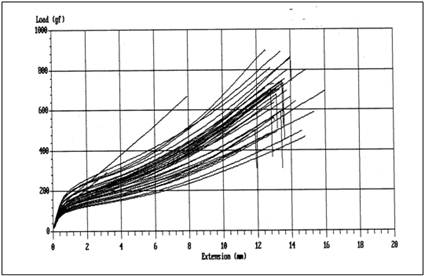Figure 1. Stress-strain curves of tested agave fibers.

2. Materials and Methods

2.1. Sampling Plan

Considering the arrangement of leaves which are disposed in a spiral-like shape, the controlling factor of ‘level’ was defined as one turn of the spiral.

The levels indicate about the age of the plant. In this manner, for a plant of 14 levels, those at the bottom of the plant (1, 2 and 3) are composed of falling or dead leaves not useable, and it is the same with level (14) in the top of the plant which include very young leaves, practically without fibers.

Thus, for the factor ‘level’, we have considered only the levels where the leaves are useable (not falling), namely the levels from 4 to 13, and in the following parts, the level N=1 corresponds to the fourth level of plant collecting. From each level, we have taken two leaves to work on, so that the total number of leaves is 20.

The fiber extraction from leaves has been carried out by degrading the leaf parenchyma in seawater.

The period of extraction depends on the level of the leaves: it is around 2 months for levels (10, 11, 12, 13) and 7 months for the rest.

Before testing, fibers were preconditioned as required in the fiber test standard, at a temperature of 20°C ± 2°C and a relative humidity of 65% ± 2%.

The fiber position in the leaf is among controlling factors we have introduced in our study. It represents the position of fibers all along the leaf (tip, middle or base parts).

In this way, the experimental design is defined by three controlling factors with their different levels.

- N = level (of leaves in the plant) 1, 2, 3, 4, 5, 6, 7, 8, 9, 10

- F = leaf (from the same level) 1, 2

- P = Position (of fibers in the leaf) 1, 2, 3

All fibers are taken from the same plant (intra plant).

Fibers samples are identified as follows: N (1 to 10), F (1 to 2), P (1 to 3)

A more detailed description of the sapling plan was reported in a previous work .

2.2. Tensile Tests and Mechanical Parameters

Tensile tests were conducted with a GAC Shirly dynamometer (type Micro 250) with the following characteristics:

Measuring accuracy (0.5% for load and 0.01 mm for elongation)

Load measurement cell : 20 N

The breakage time was fixed at 20±3 seconds as recommended by NFG07 002 French standard .

For all tests, we chose a sample gauge length of 25 mm (1 inch) as specified in ASTM D 3217 -79 American standard for the control of synthetic fibers .

Tests involved a conditioned atmosphere of 20°C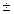2°C and 65%2% RH according to NFG00 003 French standard . Before testing, fibers were dried at ambient conditions for at least 24 hours and conditioned at 20°C2°C and 65%2% RH for at least 24 hours.

For the majority of applications, fibers endure uniaxial tensile stress. Therefore, the most important parameters to be studied are those determined by the tensile test and deduced from the stress-strain curve. The tensile tests conducted on agave fibers result in stress strain curves that are shaped as the model of figure 2.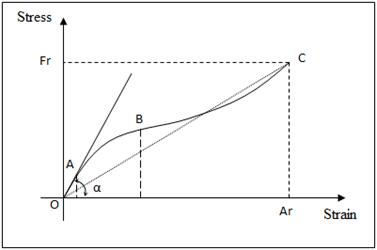Figure 2. Model of stress-strain curve for agave fiber.

The mechanical parameters we have worked on are deduced from tensile test (stress-strain curve) as follows:

Ultimate tensile strength: maximum stress supported by fiber in a tensile test and leading to failure. This parameter is usually expressed in N (Newton), but sometimes, to compare different materials regardless the dimension effect, we can use other units like Pa (Pascal). However, in textile terminology, since textile fibers present very small cross section area which couldn’t be easily determined, the ultimate tensile strength is often expressed by "tenacity" with the following formula :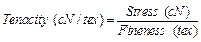Elongation at rupture: increase in fiber length (deformation) expressed in cm or mm. when fiber test length is not considered, we have to use extension expressed in percent (%) of the initial length of fiber.

Impact energy (w): the energy, expressed in Joule, for which the fiber is broken.

Initial modulus (Mo): this parameter is related to the shape of stress- strain curve, it is determined by the expression:

Mo = tg (α)

Initial modulus is the characteristic, expressed in N/tex, which informs us about the stiffness of the fiber (resistance of fiber subjected to very low stress). By this way, it permits to define the material elasticity: unlike stiff fibers, elastic fibers have a low initial modulus.

2.3. Statistical Tools

The aim of this study is to define precisely the effect of certain parameters such us the age of the leaf and the position of fibers all along the leaf. An extensive statistical study permits us to demonstrate if the variations of results are assigned only to hazardous fluctuation or to the effect of the input controlling factors considered. This study uses statistical tools such as normality test, response graph, analysis of variance (ANOVA) techniques, developed under Minitab® V14 software.

Normality test

Normality tests are used to determine if a data set is well-modeled by a normal distribution and to compute how likely it is for a random variable underlying the data set to be normally distributed . The hypotheses of this test are:

Null hypothesis = the data follow a normal law

Hypothesis= the data are not normally distributed.

In our work, we have used the probability plot test (Henry plot).

Ÿ Main effects plot

The main effects plot represents the mean value of the output responses for each level of controlling factors. This plot is basically used to compare the significance of controlling factors effects and to determine the most important factor influencing the process.

Ÿ Multivariate analysis

Multivariate analysis (MVA) is based on the statistical principle of multivariate statistics, which involves observation and analysis of more than one statistical outcome variable at a time. In design and analysis, this graphical technique is used to perform the analysis of variance across multiple dimensions while taking into account the effects of all variables (effect of interactions) on the responses .

Ÿ Interaction effects

Interaction effects represent the combined effects of factors on the dependent measure. When an interaction effect is present, the impact of one factor depends on the level of the other factor. Part of the power of ANOVA is the ability to estimate and test interaction effects. As Pedhazur and Schmelkin note, the idea that multiple effects should be studied in research rather than the isolated effects of single variables is one of the important contributions of Sir Ronald Fisher. When interaction effects are present, it means that interpretation of the main effects is incomplete or misleading.

In the digram of interaction effects, parallel Interaction results whose lines do not cross (as in the figure at left) are called "ordinal" interactions. If the slope of lines is not parallel in an ordinal interaction, the interaction effect will be significant, given enough statistical power. If the lines are parallel, then there is no interaction effect .

Ÿ Homogeneity of variances test

In some statistical tests, for example the analysis of variance, we assume that variances are equal across groups or samples (homogeneity of variances). The Bartlett test can be used to verify whether the data are normally distributed . Bartlett's test is sensitive to departures from normality. That is, if samples come from non-normal distributions, then Bartlett's test may simply be testing for non-normality. The Levene test  is an alternative to the Bartlett test that is less sensitive to departures from normality.

Ÿ Analysis of variance

The analysis of variance is a statistical test which allows to see if the controlling factor effects are more or less significant on the response. The method is based on the comparison between the real variances of the model coefficients and the variances of these same coefficients if the controlling factors have no effects .

This test consists on calculating a statistic F from the coefficients of the established model and then to compare it with F values taken from statistical tables of Snedecor law .

Ÿ If Fcal > F1% , the difference is highly significant with 1% risk of error.

Ÿ If F5% < Fcal < F1% the difference is significant with 5% risk of error.

Ÿ If Fcal < F5% the difference is not significant with 5% risk of error.

3. Results and Discussion

The effect of controlling factors on tenacity and extension is represented in figures 3, 4, 5 and 6.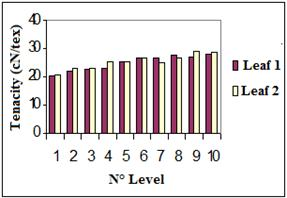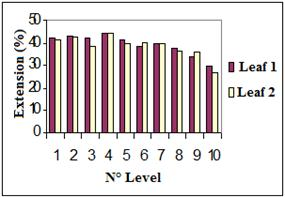Figure 3. Evolution of the tenacity and the extension as function of the factor ‘leaf’.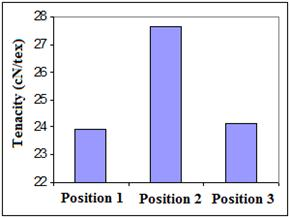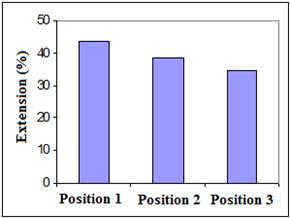Figure 4. Evolution of the tenacity and the extension as function of the factor ‘position’.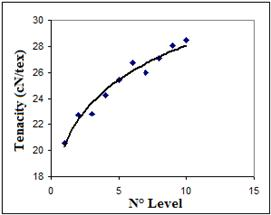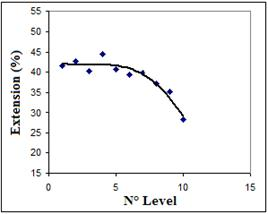Figure 5. Evolution of the tenacity and the extension as function of the factor ‘level’.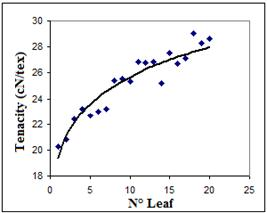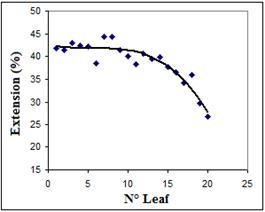Figure 6. Evolution of the tenacity and the extension as function of the factor ‘leaf position’.

Graphics related to the evolution of tenacity with the different controlling factors show an increase of this parameter which becomes more pronounced from the fifth level. This increase mode may be assigned to a beginning of biological ageing of the fibers collected from the oldest leaves. As a result, it must be noted that biological ageing and maturing is a crucial hypothesis which makes it necessary for us to select the studied leaves from the plant. Adequate precaution must be taken to prevent the collection of the youngest and the oldest leaves.

The evolution of the elongation parameter with the different studied factors, prove the results found for the tenacity, except that this time the response decreases with the increase of the factors. The downward slope is greater from the fifth level. This evolution mode of the elongation parameter correlates with the results found for the tenacity evolution, suggesting the presence of the biological maturing and ageing of fibers. Fibers provided from the youngest leaves are less extensible. This may result from the high amount of hydrogen bonds between cellulosic chains, blocking the structure of the fiber and minimizing its ability to be deformed. On the opposite side, fibers taken from the oldest leaves contain less hydrogen bonds due to the dominance of lignin and sticky substances. This explanation is illustrated in the schematization of the fiber structure as proposed in the literature  (figure 7).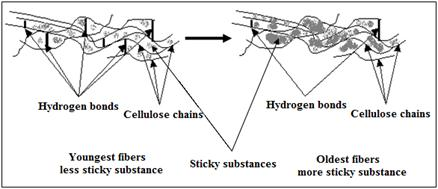Figure 7. Schematization of the leaves age effect on the presence of hydrogen bonds.

The effect of controlling factors on energy and initial modulus is represented in figures 8, 9, 10 and 11.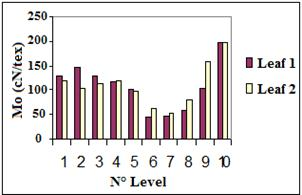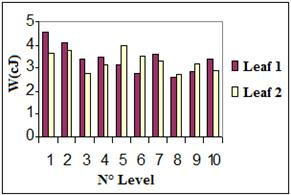Figure 8. Evolution of the initial modulus (Mo) and the impact energy (w) with the factor ‘leaf’.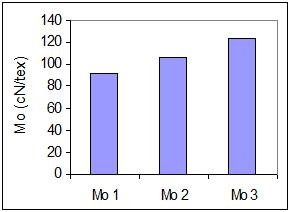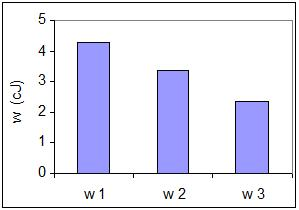Figure 9. Evolution of the initial modulus (Mo) and the impact energy (w) with the factor ‘position’.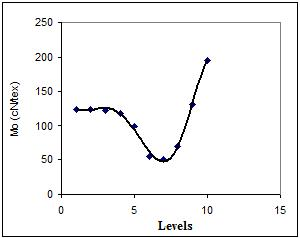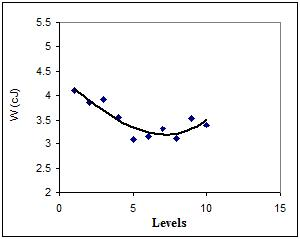Figure 10. Evolution of the initial modulus (Mo) and the impact energy (w) with the factor ‘level’.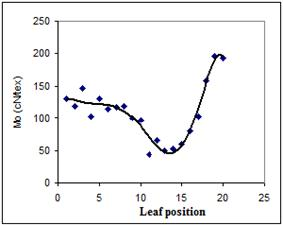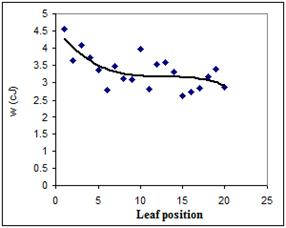Figure 11. Evolution of the initial (Mo) modulus and the impact energy (w) with the factor ‘leaf position’.

The evolution of initial modulus and impact energy with the controlling factors ‘level’ and ‘leaf’ may be explained by the molecular structure of the fiber. In fact, fibers extracted from leaves of low levels (1 to 4) exhibit approximately the same initial modulus. These fibers have reached a maturing age and in this case the amount of lignin and gums is constant at a maximum value.

For higher levels (>5), the amount of gums and lignin is less important, which leads to an increase of the number of hydrogen bonds. It is worth noting that until the 7th level, the non cellulosic materials are predominant and the stiffness of fibers decreases with the fiber age (level). From the 7th level, active sites in the fiber structure are still free and not occupied by gums and sticky substances. Thus, the presence of hydrogen bonds, in large quantities, is more defining and contributes to the blockage of the structure. For that reason, the stiffness (initial modulus) of fibers increases.

The same results were found for the evolution of impact energy which decreases with the fiber age until the 7th level, and then begins to increase. As explained above, this is due to the amount of gums and hydrogen bonds which are more or less important and define the fiber rigidity.

Ÿ Normality test

Figures 12à15 present the probability plot related to the normal law for the different studied characteristics.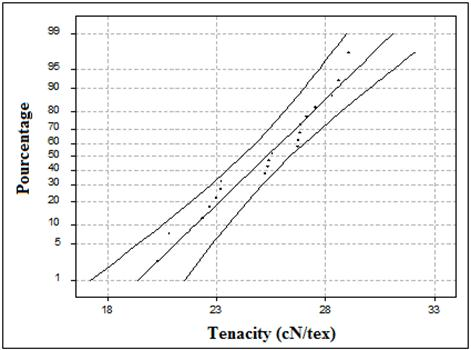Figure 12. Probability plot following a normal distribution for the tenacity.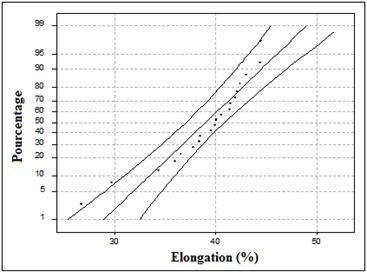Figure 13. Probability plot following a normal distribution for the elongation.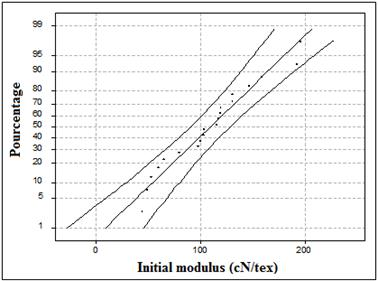Figure 14. Probability plot following a normal distribution for the initial modulus.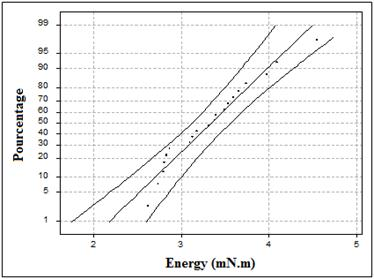Figure 15. Probability plot following a normal distribution for the impact energy.

The Henry plot (probability plot) coefficients determined from curves of figures 12à15, are gathered in table 2.

Table 2. Normality test results (Henry plot coefficient).

 Parameter Coefficient of the probability (Henry) plot Fineness 0.933 Diameter 0.940 Tenacity 0.976 Elongation 0.938 Initial modulus 0.979 Impact energy 0.978

For all studied parameters, Henry plot coefficients have high values approximately equal to 1 (» 1), which means that the experimental data could be assimilated to a normal law. Consequently, all the parameters which may affect those data have the same importance and no one is dominant.

Ÿ Analysis of the main effects

The controlling factors considered in this study are:

N = level (10 modalities)

F = Leaf (2 modalities)

P = Position (3 modalities)

The main effects of those parameters on the output parameters are presented in figures 16à19.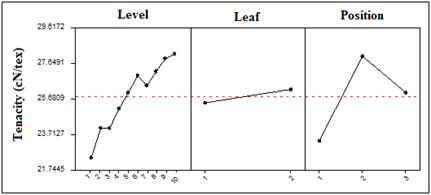Figure 16. Main effects plot for the tenacity.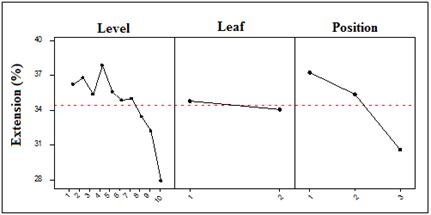Figure 17. Main effects plot for the extension.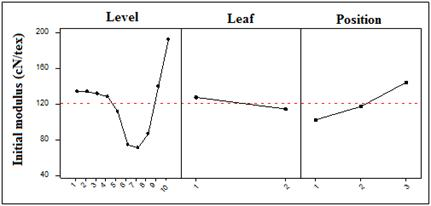Figure 18. Main effects plot for the initial modulus.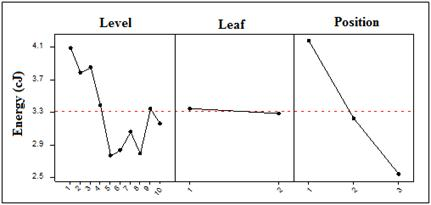Figure 19. Main effects plot for the fineness impact energy.

From graphics of figures 16à19, we deduce the importance of each controlling factor presented in table 3.

Table 3. Classification of the main effects of the controlling factors.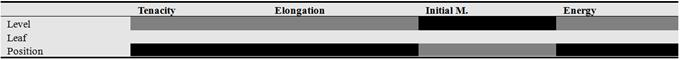With :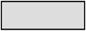Insignificant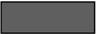Significant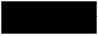Highly significant

the results of the main effects analysis show that the factor ‘leaf’ is negligible for all studied parameters , whereas the factor ‘position’ is the most influential for all parameters except for the initial modulus where factor ‘level’ is more significant. The explanation of these more or less important effects is due to the presence of lignin and gums and their effect on the fiber structure rigidity, as demonstrated previously.

Ÿ Analysis of interaction effects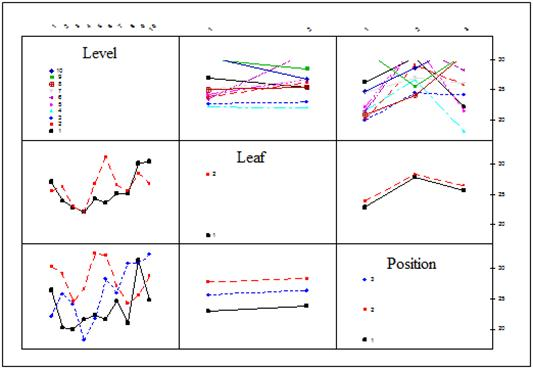Figure 20. Interaction effects relative to tenacity.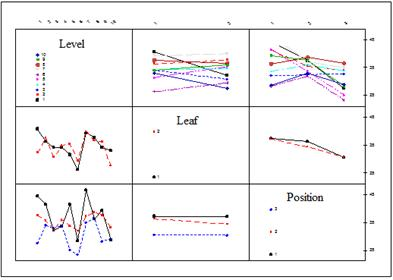Figure 21. Interaction effects relative to elongation.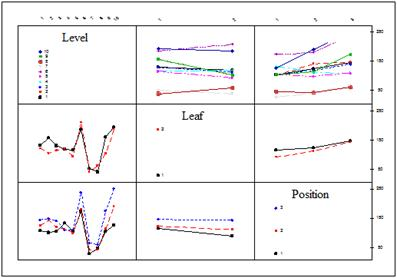Figure 22. Interaction effects relative to initial modulus.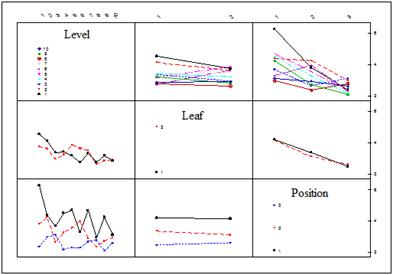Figure 23. Interaction effects relative to impact energy.

An interaction effect is present if the graphic of one factor level response depends on the other level factors, which is pictured by non parallel plots. Examining plots of figures 20à23, we can deduce the table 4 summarizing the presence and the importance of interaction effects between controlling factors.

Table 4. Identification and classification of interaction effect between two factors.

 Tenacity Elongation Initial M. Energy N ´F ´ ´ ´´´ ´´ N ´P ´´´ ´´´ ´´ ´´ F ´P - - ´ ´

With :- : the interaction is negligible or absent

´ : the interaction is present and as important as the number of x is raised.

From table 4, we note that the interaction NxP is the most important for mechanical parameters. To quantify these interaction effects, we use the analysis of variance. The results are shown in a later part.

Ÿ Equality of variances

Levene test results for the equality of variances are gathered in table 5.

Table 5. Levene test results.

 Tenacity Elongation Initial M . Energy Statistics 2.281 4.163 6.193 4.277 Probability 0.000 0.000 0.000 0.000 Conclusion Null hypothesis rejected

Ÿ Analysis of variance

The analysis of variance is the most decisive test since it allows us to classify controlling factors and to identify the most important ones. Practically, this involves the choice of leaves from the plant as well as the selection of fibers from the leaf itself.

Results of analysis of variance are presented by multivariate analysis (figures 24à27 and tables 6à9).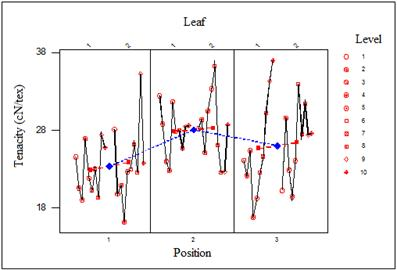Figure 24. Multivariate analysis relative to tenacity.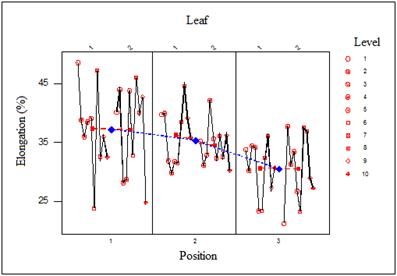Figure 25. Multivariate analysis relative to extension.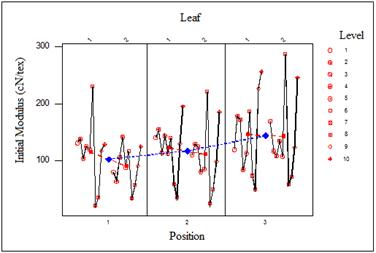Figure 26. Multivariate analysis relative to initial modulus.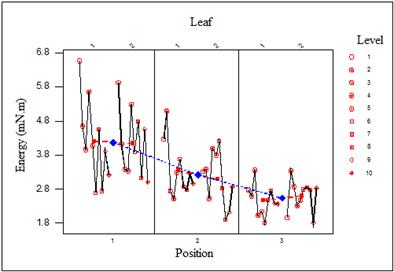Figure 27. Multivariate analysis relative to impact energy.

Table 6. Analysis of variance results for tenacity.

 DL F F (5%) F (1%) P Conclusion N 9 7.74 1.89 2.42 4.06 E –11 ´´ F 1 2.13 3.85 6.65 0.145 0 P 2 28.55 3.01 4.62 7.9 E –13 ´´ N ´F 9 3.61 1.89 2.42 0.0001 ´´ N ´P 18 7 1.62 1.95 3.6 E -17 ´´ F ´P 2 0.09 3.01 4.62 0.917 0 N ´F ´P 18 3.86 1.62 1.95 1.01 E -7 ´´

With :0 : Negligible

´ : Significant

´´ : Highly significant

Table 7. Analysis of variance results for extension.

 DL F F (5%) F (1%) P Conclusion N 9 34 1.89 2.42 0.000 ´´ F 1 3.59 3.85 6.65 0.059 ´ P 2 109.07 3.01 4.62 0.000 ´´ N ´F 9 10.9 1.89 2.42 0.000 ´´ N ´P 18 13.95 1.62 1.95 0.000 ´´ F ´P 2 2.08 3.01 4.62 0.126 0 N ´F ´P 18 10.02 1.62 1.95 0.000 ´´

Table 8. Analysis of variance results for initial modulus.

 DL F F (5%) F (1%) P Conclusion N 9 89.57 1.89 2.42 7.2 E -126 ´´ F 1 16.6 3.85 6.65 4.9 E -5 ´´ P 2 54.9 3.01 4.62 1.7 E -23 ´´ N ´F 9 6.25 1.89 2.42 1.17 E -8 ´´ N ´P 18 5.66 1.62 1.95 4.6 E -13 ´´ F ´P 2 3.49 3.01 4.62 0.031 ´ N ´F ´P 18 8.34 1.62 1.95 2.6 E -21 ´´

Table 9. Analysis of variance results for impact energy.

 DL F F (5%) F (1%) P Conclusion N 9 7.72 1.89 2.42 4.3 E -11 ´´ F 1 0.32 3.85 6.65 0.569 0 P 2 88.61 3.01 4.62 1.7 E -13 ´´ N ´F 9 2.78 1.89 2.42 0.003 ´´ N ´P 18 5.91 1.62 1.95 7.9 E -14 ´ F ´P 2 1.23 3.01 4.62 0.293 0 N ´F ´P 18 2.44 1.62 1.95 0.001 ´´

Table 10. Recapitulation of ANOVA results.With: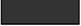Highly significant;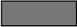Significant;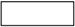Negligible

Table 10 summarizes the main results of intra-plant variability study on agave fiber mechanical properties. The assessment of controlling factors effects permits us practically to orientate the collecting and the choice of fibers for future applications.

Except for elongation and initial modulus parameters, the factor ‘leaf’ is negligible for all other characteristics. This means that fibers taken from different leaves at the same level of a plant have approximately much close properties. However, the factor ‘position’ is the most crucial one, which means that fibers located at different positions in the leaf (tip, middle or base) exhibit different properties.

Besides, the factor ‘level’ is much important, given that fibers belonging to inferior levels of the plant (oldest leaves) have different properties compared to those selected from the top of the plant (youngest leaves). It’s worth noting that fibers taken from the middle part of the plant give the best mechanical properties in terms of tenacity and stiffness. Thus, the top and the bottom parts of the plant have to be avoided when collecting leaves.

The 2-order interactions have significant effects for mechanical properties. Consequently, the simultaneous changes of factors levels may alter mechanical characteristics.

4. Conclusions

The determination of mechanical properties for agave fibers shows a great dispersion in results. The aim of this paper was to quantify, materially this variability which can be assigned to the extraction mode. As a matter of fact, fibers may be extracted from different parts of the plant and of the same leaf.

The Design of experiment DoE techniques, and other statistical analyses were used by varying the factors of interest in a full factorial design to assess the main effects and the interactions of some factors influencing the fiber mechanical properties.

The controlling factors were chosen as follows:

- ‘Level’ of leaves in the plant which informs about the age of the pant,

- ‘Leaf’ taken from the same level

- ‘Position’ of fibers in the leaf, that is related to the maturity of fibers.

All fibers are taken from the same plant.

The results of the main effects analysis show that the factor ‘leaf’ is negligible, in other words, fibers taken from different leaves of the same level have approximately much close properties. On the other hand, the factor ‘position’ is the most influential for the different studied parameters which means that fibers located at different positions in the leaf (tip, middle or base) have not necessarily the same resistance and stiffness values.

The factor ‘level’ is significant, given that fibers belonging to inferior levels of the plant (oldest leaves) have different mechanical properties compared to those selected from the top of the plant (youngest leaves). In fact, it was noted that fiber provided from the youngest leaves are more resistant and less extensible. This may result from the high amount of hydrogen bonds between cellulosic chains, blocking the structure of the fiber and minimizing its ability to be deformed. On the opposite side, fibers taken from the oldest leaves contain less hydrogen bonds due to the dominance of lignin and sticky substances, and consequently they are less resistant and more extensible.

References

1. S.Msahli,F.SakliandJ. Y.Drean, Study of Textile Potential of Fibers Extracted From Tunisian Agave americana L., AUTEX Res. J. 6(1), 9-13, 2006.
2. Y.Chaabouni,2005. Cractérisation de la microstructure de la fibre d’Agave americana L., contribution à l’étude de composites renforcés par des fibres d’agave, PhD thesis, Université de Haute Alsace, Mulhouse, France.
3. S. Msahli,Etude du potentiel textile des fibres d’Agave americana L., Ph.D. thesis, University of Mulhouse, France, 2000.
4. A.El Oudiani,S. Msahli, F.Sakliand J. Y. Drean, Variability in Fineness Parameter of Agave Americana L. Fiber "Intra-Plant Study",Accepted by advanced Materials (2015).
5. Détermination de la force et de l’allongement de rupture par traction, méthode simplifiée, NFG 07-002, Paris: AFNOR, 1985.
6. Test method for breaking tenacity of man-made textile fibers in loop or knot configuration, ASTM 3217 – 1779, USA: ASTM Norms, 1979
7. Fibres et fils – analyse chimique, Atmosphère normale de référence et atmosphère normale de conditionnement et d’essais des textiles, NF G 00 – 003 (Août 1970), Paris : AFNOR, 1970, page 44
8. S. F. Arnold, Mathematical Statistics, Prentice-Hall, 1990, pages 383 – 384.
9. J. J. Filliben, The Probability Plot Correlation Coefficient Test for Normality Technometrics, 1975, Vol. 17, pages 111.
10. R. B. Daugostino & M. A. Stevens, Goodness of Fit Techniques, Marcel Dekker, 1986
11. A. Agreshi, Categorial Data Analysis, John Wiley and Sons, 1990.
12. C. R. Hicks, Fundamental concepts in the design of experiments, 3rd Ed, CBC College Publishing, 1982.
13. M. B. Brown & A. B. Forsythe, Journal of the American Statistical Association, 1974, N° 69, pages 364 – 367.
14. H. Levene, Contributions to Probability and Statistics, Stanford University Press, CA, 1960, pages 278 – 292.
15. G. A. Milliken & D. E. Johnson, Analysis of Messy Data, Vol. 1: Designed Experiments, Van Reinhold, 1984.
16. P. R. Nelson, A comparison of sample seizes for the analysis of means and analysis of variances, Journal of Quality Technology, 1983, N° 15, pages 33 – 39.
17. R. A. Olshen, The conditional level of the F-test, Journal of the American Association, 1973, N° 68, pages 692 – 698.
18. D. C. Prevorsek et Y. D. Kwon, J. Macromol. Scien – Phys., B 12 (4), 1976, pages 447 – 485.

 Contents 1. 2. 2.1. 2.2. 2.3. 3. 4.
Article ToolsAbstractPDF(526K)